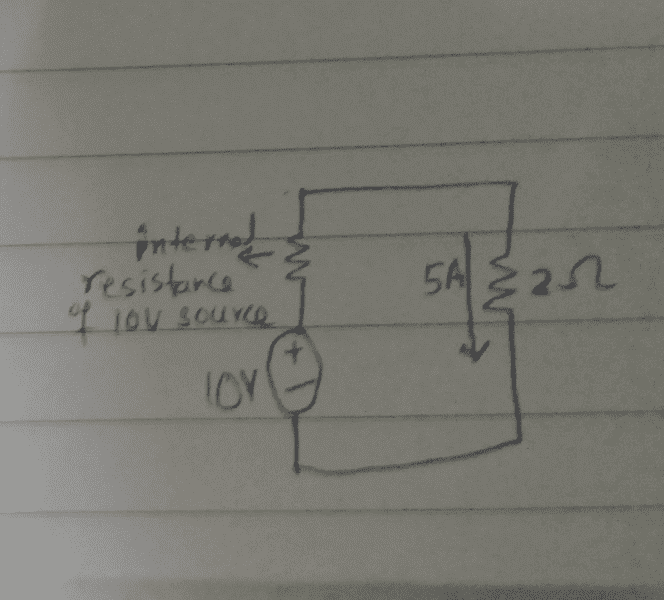# Voltage drop in the internal resistance of a voltage source

• Engineer1
In summary, when analyzing a circuit with a practical voltage source, it is important to consider the voltage drop in the internal resistance of the source. This voltage drop will depend on the value of the internal resistance, and the remaining voltage will appear at the load. The amount of current that flows through the load will depend on the voltage drop across it, and the source and load resistances can be calculated using Ohm's Law. However, the concept of internal resistance is only an approximation and may not always accurately explain the voltage drop under load. It is also important to note that the current flow through the load will determine the voltage drop in the source's internal resistance.f

#### Engineer1

Gold Member
In this circuit,first there will be voltage drop in the internal resistance of the voltage source.Mostly,the voltage source is assumed ideal in circuits,however,here a practical voltage source is assumed.
So,there will be voltage drop in the internal resistance of the voltage source which will depend on the value of the internal resistance and the remaining voltage will appear at 2 ohms load.The amount of current which flows through that 2 ohms load will depend on the value of the value of the voltage drop across the 2 ohms load.The more the potential difference across the 2 ohms load.the more current will flow.Is this right?

It is not the other way round that the value of the voltage drop in internal resistance is decided by the current which flows through 2 ohms load? First,there should be voltage drop in internal resistance and the remaining voltage should appear at 2 ohms load resistance and the amount of current in 2 ohms will depend on that voltage appearing across 2 ohms.Is that right.#### Attachments

You have a voltage source and a total resistance. It is irrelevant that some of the resistance is internal to the voltage source. Use Ohms Law.

You have a voltage source and a total resistance. It is irrelevant that some of the resistance is internal to the voltage source. Use Ohms Law.
Practical voltage source has internal resistance as well.Mostly,for circuit analysis ideal voltage source is taken and that internal resistance is not considered.

Practical voltage source has internal resistance as well.Mostly,for circuit analysis ideal voltage source is taken and that internal resistance is not considered.
You're not listening to what I said. Why do you think I said you have a TOTAL resistance?

•davenn, Engineer1 and Bystander
You're not listening to what I said. Why do you think I said you have a TOTAL resistance?
yes,in series the resistances add and accordingly the total voltage drop and thus the current flow.

In series resistances are added,this is what is done for calculations.But it is the same thing if one finds voltage drop at each resistance separately and thus the current flow.
I heard somewhere that the current flow in load resistance decides the voltage drop in internal resistance of voltage source.It seemed confusing.

You're not listening to what I said. Why do you think I said you have a TOTAL resistance?
Hey,thank you for your response.In series resistances are added,this is what is done for calculations.But it is the same thing if one finds voltage drop at each resistance separately and thus the current flow.
I heard somewhere that the current flow in load resistance decides the voltage drop in internal resistance of voltage source.It seemed confusing.
Yeah, when you're starting out it can ALL be a bit confusing but it gets easy pretty quickly.

You always need two measurements for this; one with no load to give the emf and one with a known load resistance. That gives two equations and you can solve for the internal resistance.
But remember: the term 'internal resistance' is only a convenient approximation. Many sources will have a voltage drop under load which cannot be explained by a constant equivalent series resistance inside them. The effect is very often non-linear and can be time dependent if, for example, a battery warms up under load.

In series resistances are added,this is what is done for calculations.But it is the same thing if one finds voltage drop at each resistance separately and thus the current flow.

Yes. If you measure the voltage across the 2R then you know the current flowing in it. Since everything is in series the current flowing through the source and source resistance is the same.

I heard somewhere that the current flow in load resistance decides the voltage drop in internal resistance of voltage source.It seemed confusing.

Correct. The lower the load resistance the greater the current flowing and the greater the voltage drop across the source resistor. If you replaced the load with a short circuit (0R) then all of the source voltage would be across the source internal resistor. Beware this may not be safe to do in practice, the current might be dangerously high.

•Engineer1